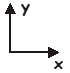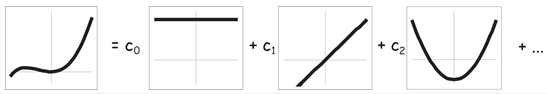# 15: Reduction of representations II

•• Contributed by Claire Vallance
• Professor of Physical Chemistry (Department of Chemistry) at University of Oxford

By making maximum use of molecular symmetry, we often greatly simplify problems involving molecular properties. For example, the formation of chemical bonds is strongly dependent on the atomic orbitals involved having the correct symmetries. To make full use of group theory in the applications we will be considering, we need to develop a little more ‘machinery’. Specifically, given a basis set (of atomic orbitals, for example) we need to find out:

1. How to determine the irreducible representations spanned by the basis functions
2. How to construct linear combinations of the original basis functions that transform as a given irreducible representation/symmetry species.

It turns out that both of these problems can be solved using something called the ‘Great Orthogonality Theorem’ (GOT for short). The GOT summarizes a number of orthogonality relationships implicit in matrix representations of symmetry groups, and may be derived in a somewhat qualitative fashion by considering these relationships in turn.

Some of you might find the next section a little hard going. In it, we will derive two important expressions that we can use to achieve the two goals we have set out above. It is not important that you understand every step in these derivations; they have mainly been included just so you can see where the equations come from. However, you will need to understand how to use the results. Hopefully you will not find this too difficult once we’ve worked through a few examples.

## General concepts of Orthogonality

You are probably already familiar with the geometric concept of orthogonality. Two vectors are orthogonal if their dot product (i.e. the projection of one vector onto the other) is zero. An example of a pair of orthogonal vectors is provided by the $$\textbf{x}$$ and $$\textbf{y}$$ Cartesian unit vectors.

$\textbf{x}, \textbf{y} = 0\label{15.1}$A consequence of the orthogonality of $$\textbf{x}$$ and $$\textbf{y}$$ is that any general vector in the $$xy$$ plane may be written as a linear combination of these two basis vectors.

$\textbf{r} = a\textbf{x} + b\textbf{y} \label{15.2}$Mathematical functions may also be orthogonal. Two functions, $$f_1(x)$$ and $$f_2(x)$$, are defined to be orthogonal if the integral over their product is equal to zero i.e.

$\int f_1(x) f_2(x) dx = \delta_{12}$

This simply means that there must be ‘no overlap’ between orthogonal functions, which is the same as the orthogonality requirement for vectors, above. In the same way as for vectors, any general function may be written as a linear combination of a suitably chosen set of orthogonal basis functions. For example, the Legendre polynomials $$P_n(x)$$ form an orthogonal basis set for functions of one variable $$x$$.

$f(x) = \sum_n c_n P_n(x) \label{15.3}$## Orthogonality relationships in Group Theory

The irreducible representations of a point group satisfy a number of orthogonality relationships:

1. If corresponding matrix elements in all of the matrix representatives of an irreducible representation are squared and added together, the result is equal to the order of the group divided by the dimensionality of the irreducible representation. i.e.

$\sum _g \Gamma_k(g)_{ij} \Gamma_k(g)_{ij} = \dfrac{h}{d_k} \label{15.4}$

where $$k$$ labels the irreducible representation, $$i$$ and $$j$$ label the row and column position within the irreducible representation, $$h$$ is the order of the group, and $$d_k$$ is the order of the irreducible representation. e.g. The order of the group $$C_{3v}$$ is 6. If we apply the above operation to the first element in the 2x2 ($$E$$) irreducible representation derived in Section 12, the result should be equal to $$\dfrac{h}{d_k}$$ = $$\dfrac{6}{2}$$ = 3. Carrying out this operation gives:

$(1)^2 + (-\dfrac{1}{2})^2 + (-\dfrac{1}{2})^2 + (1)^2 + (-\dfrac{1}{2})^2 +(-\dfrac{1}{2})^2 = 1 + \dfrac{1}{4} + \dfrac{1}{4} + 1 + \dfrac{1}{4} + \dfrac{1}{4} = 3 \label{15.5}$

2. If instead of summing the squares of matrix elements in an irreducible representation, we sum the product of two different elements from within each matrix, the result is equal to zero. i.e.

$\sum _g \Gamma_k(g)_{ij} \Gamma_k(g)_{i'j'} = 0 \label{15.6}$

where $$i \neq i'$$ and/or $$j \neq j'$$. E.g. if we perform this operation using the two elements in the first row of the 2D irreducible representation used in 1, we get:

$(1)(0) + (-\dfrac{1}{2})(\dfrac{\sqrt{3}}{2}) + (-\dfrac{1}{2})(-\dfrac{\sqrt{3}}{2}) + (1)(0) + (-\dfrac{1}{2})(\dfrac{\sqrt{3}}{2}) + (-\dfrac{1}{2})(-\dfrac{\sqrt{3}}{2}) = 0 + \dfrac{\sqrt{3}}{4} - \dfrac{\sqrt{3}}{4} + 0 - \dfrac{\sqrt{3}}{4} + \dfrac{\sqrt{3}}{4} = 0 \label{15.7}$

3. If we sum the product of two elements from the matrices of two different irreducible representations $$k$$ and $$m$$, the result is equal to zero. i.e.

$\sum_g \Gamma_k(g)_{ij} \Gamma_m(g)_{i'j'} = 0 \label{15.8}$

where there is now no restriction on the values of the indices $$i$$, $$i'$$, $$j$$, $$j'$$ (apart from the rather obvious restriction that they must be less than or equal to the dimensions of the irreducible representation). e.g. Performing this operation on the first elements of the $$A_1$$ and $$E$$ irreducible representations we derived for $$C_{3v}$$ gives:

$(1)(1) + (1)(-\dfrac{1}{2}) + (1)(-\dfrac{1}{2}) + (1)(1) + (1)(-\dfrac{1}{2}) + (1)(-\dfrac{1}{2}) = 1 - \dfrac{1}{2} - \dfrac{1}{2} + 1 - \dfrac{1}{2} - \dfrac{1}{2} = 0 \label{15.9}$

We can combine these three results into one general equation, the Great Orthogonality Theorem$$^4$$.

$\sum_g \Gamma_k(g)_{ij} \Gamma_m(g)_{i'j'} = \dfrac{h}{\sqrt{d_kd_m}} \delta_{km} \delta_{ii'} \delta_{jj'} \label{15.10}$

For most applications we do not actually need the full Great Orthogonality Theorem. A little mathematical trickery transforms Equation $$\ref{15.10}$$ into the ‘Little Orthogonality Theorem’ (or LOT), which is expressed in terms of the characters of the irreducible representations rather than the irreducible representations themselves.

$\sum_g \chi_k(g) \chi_m(g) = h\delta_{km} \label{15.11}$

Since the characters for two symmetry operations in the same class are the same, we can also rewrite the sum over symmetry operations as a sum over classes.

$\sum_C n_C \chi_k(C) \chi_m(C) = h \delta_{km} \label{15.12}$

where $$n_C$$ is the number of symmetry operations in class $$C$$.

In all of the examples we’ve considered so far, the characters have been real. However, this is not necessarily true for all point groups, so to make the above equations completely general we need to include the possibility of imaginary characters. In this case we have:

$\sum_C n_C \chi_k^*(C) \chi_m(C) = h \delta_{km} \label{15.13}$

where $$\chi_k^*(C)$$ is the complex conjugate of $$\chi_k(C)$$. Equation $$\ref{15.13}$$ is of course identical to Equation $$\ref{15.12}$$ when all the characters are real.

## Using the LOT to Determine the Irreducible Representations Spanned by a Basis

In Section $$12$$ we discovered that we can often carry out a similarity transform on a general matrix representation so that all the representatives end up in the same block diagonal form. When this is possible, each set of submatrices also forms a valid matrix representation of the group. If none of the submatrices can be reduced further by carrying out another similarity transform, they are said to form an irreducible representation of the point group. An important property of matrix representatives is that their character is invariant under a similarity transform. This means that the character of the original representatives must be equal to the sum of the characters of the irreducible representations into which the representation is reduced. e.g. if we consider the representative for the $$C_3^-$$ symmetry operation in our $$NH_3$$ example, we have:

$\begin{array}{ccccc} \begin{pmatrix} 1 & 0 & 0 & 0 \\ 0 & 0 & 0 & 1 \\ 0 & 1 & 0 & 0 \\ 0 & 0 & 1 & 0 \end{pmatrix} & \begin{array}{c} \text{similarity transform} \\ \longrightarrow \end{array} & \begin{pmatrix} 1 & 0 & 0 & 0 \\ 0 & 1 & 0 & 0 \\ 0 & 0 & -\dfrac{1}{2} & -\dfrac{\sqrt{3}}{2} \\ 0 & 0 & \dfrac{\sqrt{3}}{2} & -\dfrac{1}{2} \end{pmatrix} & = & (1) \otimes (1) \otimes \begin{pmatrix} -\dfrac{1}{2} -\dfrac{\sqrt{3}}{2} \\ \dfrac{\sqrt{3}}{2} -\dfrac{1}{2} \end{pmatrix} \\ \chi = 1 & & \chi = 1 & & \chi = 1 + 1 - 1 = 1 \end{array} \label{15.14}$

It follows that we can write the characters for a general representation $$\Gamma(g)$$ in terms of the characters of the irreducible representations $$\Gamma_k(g)$$ into which it can be reduced.

$\chi(g) = \sum_k a_k \chi_k(g) \label{15.15}$

where the coefficients $$a_k$$ in the sum are the number of times each irreducible representation appears in the representation. This means that in order to determine the irreducible representations spanned by a given basis. all we have to do is determine the coefficients $$a_k$$ in the above equation. This is where the Little Orthogonality Theorem comes in handy. If we take the LOT in the form of Equation $$\ref{15.15}$$, and multiply each side through by $$a_k$$, we get

$\Sigma_g a_k \chi_k(g) \chi_m(g) = h a_k \delta_{km} \label{15.16}$

Summing both sides of the above equation over $$k$$ gives

$\Sigma_g \Sigma_k a_k \chi_k(g) \chi_m(g) = h \Sigma_k a_k \delta_{km} \label{15.17}$

We can use Equation $$\ref{15.15}$$ to simplify the left hand side of this equation. Also, the sum on the right hand side reduces to $$a_m$$ because $$\delta{km}$$ is only non-zero (and equal to $$1$$) when $$k$$ = $$m$$

$\Sigma_g \chi(g) \chi_m(g) = h a_m \label{15.18}$

Dividing both sides through by $$h$$ (the order of the group), gives us an expression for the coefficients $$a_m$$ in terms of the characters $$\chi(g)$$ of the original representation and the characters $$\chi_m(g)$$ of the $$m^{th}$$ irreducible representation.

$a_m = \dfrac{1}{h} \Sigma_g \chi(g) \chi_m(g) \label{15.19}$

We can of course write this as a sum over classes rather than a sum over symmetry operations.

$a_m = \dfrac{1}{h} \Sigma_C n_C \chi(g) \chi_m(g) \label{15.20}$

As an example, in Section $$12$$ we showed that the matrix representatives we derived for the $$C_{3v}$$ group could be reduced into two irreducible representations of $$A_1$$ symmetry and one of $$E$$ symmetry. i.e. $$\Gamma$$ = 2$$A_1$$ + $$E$$. We could have obtained the same result using Equation $$\ref{15.20}$$). The characters for our original representation and for the irreducible representations of the $$C_{3v}$$ point group ($$A_1$$, $$A_2$$ and $$E$$) are given in the table below.

$\begin{array}{llll} \hline C_{3v} & E & 2C_3 & 3\sigma_v \\ \hline \chi & 4 & 1 & 2 \\ \hline \chi(A_1) & 1 & 1 & 1 \\ \chi(A_2) & 1 & 1 & -1 \\ \chi(E) & 2 & -1 & 0 \\ \hline \end{array} \label{15.21}$

From Equation $$\ref{15.20}$$, the number of times each irreducible representation occurs for our chosen basis $$\begin{pmatrix} s_n, s_1, s_2, s_3 \end{pmatrix}$$ is therefore

$\begin{array}{l} a(A_1) = \dfrac{1}{6}(1x4x1 + 2x1x1 + 3x2x1) = 2 \\ a(A_2) = \dfrac{1}{6}(1x4x1 + 2x1x1 + 3x2x-1) = 0 \\ a(E) = \dfrac{1}{6}(1x4x2 + 2x1x-1 + 3x2x0) = 1 \end{array} \label{15.22}$

i.e. Our basis is spanned by $$2A_1$$ + $$E$$, as we found before.

$$^4$$The $$\delta_{ij}$$ appearing in Equation $$\ref{15.10}$$ are called Dirac delta functions. They are equal to $$1$$ if $$i$$ = $$j$$ and $$0$$ otherwise.